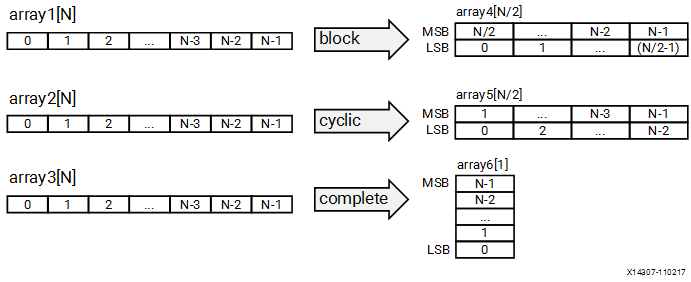# pragma HLS array_reshape - 2021.2 English

Document ID
UG1399
Release Date
2021-12-15
Version
2021.2 English

## Description

Important: `Array_Partition` and `Array_Reshape` pragmas and directives are not supported for `M_AXI` Interfaces on the top-level function. Instead you can use the `hls::vector` data types as described in Vector Data Types.

The ARRAY_RESHAPE pragma reforms the array with vertical remapping and concatenating elements of arrays by increasing bit-widths. This reduces the number of block RAM consumed while providing parallel access to the data. This pragma creates a new array with fewer elements but with greater bit-width, allowing more data to be accessed in a single clock cycle.

Given the following code:

``````void foo (...) {
int array1[N];
int array2[N];
int array3[N];
#pragma HLS ARRAY_RESHAPE variable=array1 type=block factor=2 dim=1
#pragma HLS ARRAY_RESHAPE variable=array2 type=cycle factor=2 dim=1
#pragma HLS ARRAY_RESHAPE variable=array3 type=complete dim=1
...
}``````

The ARRAY_RESHAPE pragma transforms the arrays into the form shown in the following figure.

Figure 1. ARRAY_RESHAPE Pragma## Syntax

Place the pragma in the C source within the region of a function where the array variable is defines.

``#pragma HLS array_reshape variable=<name> \type=<type>  factor=<int>  dim=<int>``

Where:

`variable=<name>`
Required argument that specifies the array variable to be reshaped.
`type=<type>`
Optionally specifies the partition type. The default type is `complete`. The following types are supported:
`cyclic`
Cyclic reshaping creates smaller arrays by interleaving elements from the original array. For example, if `factor=3` is used, element 0 is assigned to the first new array, element 1 to the second new array, element 2 is assigned to the third new array, and then element 3 is assigned to the first new array again. The final array is a vertical concatenation (word concatenation, to create longer words) of the new arrays into a single array.
`block`
Block reshaping creates smaller arrays from consecutive blocks of the original array. This effectively splits the array into `<N>` equal blocks where `<N>` is the integer defined by `factor=`, and then combines the `<N>` blocks into a single array with `word-width*N`.
`complete`
Complete reshaping decomposes the array into temporary individual elements and then recombines them into an array with a wider word. For a one-dimension array this is equivalent to creating a very-wide register (if the original array was N elements of M bits, the result is a register with `N*M` bits). This is the default type of array reshaping.
`factor=<int>`
Specifies the amount to divide the current array by (or the number of temporary arrays to create). A factor of 2 splits the array in half, while doubling the bit-width. A factor of 3 divides the array into three, with triple the bit-width.
Important: For complete type partitioning, the factor is not specified. For block and cyclic reshaping, the `factor=` is required.
`dim=<int>`
Specifies which dimension of a multi-dimensional array to partition. Specified as an integer from 0 to `<N>`, for an array with `<N>` dimensions:
• If a value of 0 is used, all dimensions of a multi-dimensional array are partitioned with the specified type and factor options.
• Any non-zero value partitions only the specified dimension. For example, if a value 1 is used, only the first dimension is partitioned.
`object`
A keyword relevant for container arrays only. When the keyword is specified the ARRAY_RESHAPE pragma applies to the objects in the container, reshaping all dimensions of the objects within the container, but all dimensions of the container itself are preserved. When the keyword is not specified the pragma applies to the container array and not the objects.

## Example 1

Reshapes an 8-bit array with 17 elements, `AB`, into a new 32-bit array with five elements using block mapping.

``#pragma HLS array_reshape variable=AB type=block factor=4``
Tip: `factor=4` indicates that the array should be divided into four; this means that 17 elements are reshaped into an array of five elements, with four times the bit-width. In this case, the last element, `AB`, is mapped to the lower eight bits of the fifth element, and the rest of the fifth element is empty.

## Example 2

Reshapes the two-dimensional array `AB` into a new array of dimension , in which dimension 2 has twice the bit-width.

``#pragma HLS array_reshape variable=AB type=block factor=2 dim=2``

## Example 3

Reshapes the three-dimensional 8-bit array, `AB` in function `func`, into a new single element array (a register), 128-bits wide (4×2×2×8).

``#pragma HLS array_reshape variable=AB type=complete dim=0``
Tip: `dim=0` means to reshape all dimensions of the array.

## Example 4

Partitioned arrays can be addressed in your code by the new structure of the array, as shown in the following code example;

``````struct SS
{
int x[N];
int y[N];
};

int top(SS *a, int b, SS &c) {
#pragma HLS array_reshape type=complete dim=0 variable=b
#pragma HLS interface mode=ap_memory port=b
``````